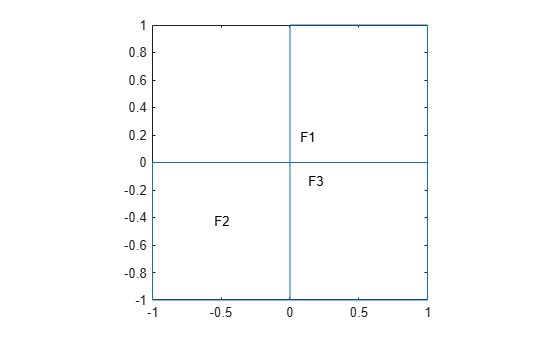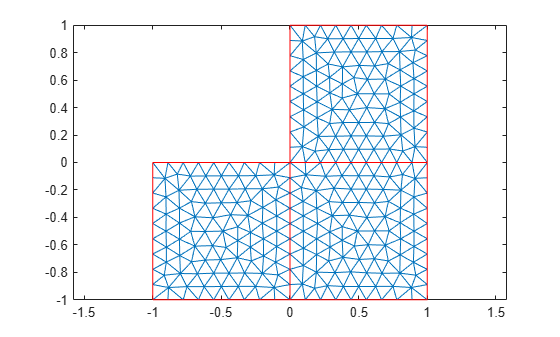Documentation

# area

Area of 2-D mesh elements

## Syntax

``A = area(mesh)``
``[A,AE] = area(mesh)``
``A = area(mesh,elements)``

## Description

example

````A = area(mesh)` returns the area `A` of the entire mesh.```

example

````[A,AE] = area(mesh)` also returns a row vector `AE` containing areas of each individual element of the mesh.```

example

````A = area(mesh,elements)` returns the combined area of the specified elements of the mesh.```

## Examples

collapse all

Generate a 2-D mesh and find its area.

Create a PDE model.

`model = createpde;`

Include the geometry of the built-in function `lshapeg`. Plot the geometry.

```geometryFromEdges(model,@lshapeg); pdegplot(model,'FaceLabels','on')```Generate a mesh and plot it.

```mesh = generateMesh(model); figure pdemesh(model)```Compute the area of the entire mesh.

`ma = area(mesh)`
```ma = 3.0000 ```

Generate a 2-D mesh and find the area of each element.

Create a PDE model.

`model = createpde;`

Include the geometry of the built-in function `lshapeg`. Plot the geometry.

```geometryFromEdges(model,@lshapeg); pdegplot(model,'FaceLabels','on')```Generate a mesh and plot it.

```mesh = generateMesh(model); figure pdemesh(model)```Compute the area of the entire mesh and the area of each individual element of the mesh. Display the areas of the first 5 elements.

```[ma,mi] = area(mesh); mi(1:5)```
```ans = 1×5 0.0047 0.0054 0.0053 0.0048 0.0061 ```

Find the combined area of the elements associated with a particular face of a 2-D mesh.

Create a PDE model.

`model = createpde;`

Include the geometry of the built-in function `lshapeg`. Plot the geometry.

```geometryFromEdges(model,@lshapeg); pdegplot(model,'FaceLabels','on')```Generate a mesh and plot it.

```mesh = generateMesh(model); figure pdemesh(model)```Find the elements associated with face 1 and compute the total area of these elements.

```Ef1 = findElements(mesh,'region','Face',1); maf1 = area(mesh,Ef1)```
```maf1 = 1.0000 ```

Find how much of the total mesh area belongs to these elements. Return the result as a percentage.

`maf1_percent = maf1/area(mesh)*100`
```maf1_percent = 33.3333 ```

## Input Arguments

collapse all

Mesh object, specified as the `Mesh` property of a `PDEModel` object or as the output of `generateMesh`.

Example: `model.Mesh`

Element IDs, specified as a positive integer or a matrix of positive integers.

Example: `[10 68 81 97 113 130 136 164]`

## Output Arguments

collapse all

Area of the entire mesh or the combined area of the specified elements of the mesh, returned as a positive number.

Areas of individual elements, returned as a row vector of positive numbers.

## See Also

### Topics

#### Introduced in R2018a

##### Support평가판 신청